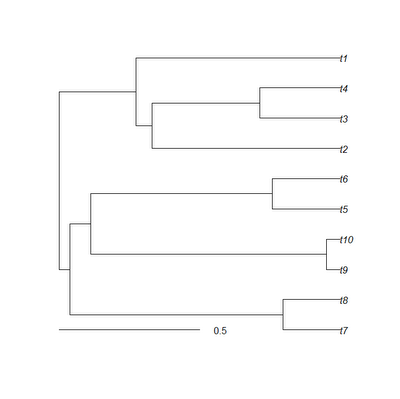## Saturday, March 3, 2012

### New version of treeSlice

The phytools function treeSlice cuts a phylogeny at a particular height above the root and returns all non-trivial subtrees (that is, subtrees with two or more taxa). A user requested that I add the capacity to return trivial as well as non-trivial subtrees. This is straightforward, but it cannot be done using the ape function extract.clade, because that only permits the extraction of trees with two or more tips. Instead, for subtrees with only one tip we create the "phylo" object directly. Here is how we do it:

for(i in 1:length(nodes)){
if(nodes[i]>length(tree\$tip)){
trees[[i]]\$root.edge<-H[which(tree\$edge[,2]==nodes[i]),2] -slice
} else {
z<-list(edge=matrix(c(2,1),1,2), edge.length=H[which(tree\$edge[,2]==nodes[i]),2]-slice, tip.label=tree\$tip.label[nodes[i]],Nnode=1L)
class(z)<-"phylo"; trees[[i]]<-z
}
}

Seems to work fine. The default performance of treeSlice is to return only non-trivial subtrees. To return trivial subtrees, we set the new argument trivial=TRUE. So, for instance:

> set.seed(1)
> tree<-pbtree(n=10,scale=1)> trees<-treeSlice(tree,0.5,trivial=T)
> str(trees)
Class "multiPhylo"
List of 6
\$ :List of 5
..\$ edge       : num [1:2, 1:2] 3 3 1 2
..\$ edge.length: num [1:2] 0.204 0.204
..\$ tip.label  : chr [1:2] "t7" "t8"
..\$ Nnode      : int 1
..\$ root.edge  : num 0.296
..- attr(*, "class")= chr "phylo"
...
\$ :List of 4
..\$ edge       : num [1, 1:2] 2 1
..\$ edge.length: num 0.5
..\$ tip.label  : chr "t2"
..\$ Nnode      : int 1
..- attr(*, "class")= chr "phylo"
...

That's it.

I have posted a new version of phytools with this function. You can download it here and install from source.

1.I tried to use the treeSlice function to split a tree into subtrees. I also want all of the 'trivial' subtrees consisting of a single leaf. When the slice parameter is set to a value higher than the distance from the root to some nodes, those nodes do not show up in any of the subtrees returned by treeSlice. Here is some example code that illustrates this:

require("phylotools")
# generate random trees with N nodes until you find a tree the yields fewer tips/leafs than
# you expected when you run the treeSlice command:

set.seed(120984)
N = 20;
cutoff = 0.5;
rt1=rtree(N);
n2 = sum(unlist(lapply(treeSlice(rt1,cutoff, trivial = T), function(x) length(x\$tip.label))))
while(N-n2 < 3)
{
rt1=rtree(N);
n2 = sum(unlist(lapply(treeSlice(rt1,cutoff, trivial = T), function(x) length(x\$tip.label))))
}

plot(rt1)
# get names of missing leafs:
setdiff(paste0('t', 1:N), unlist(lapply(treeSlice(rt1,cutoff, trivial = T), function(x) x\$tip.label)))

1.Thanks for the question.

I have posted a clarification here. Let me know if you have any questions.

- Liam

2.Thank you for the clarification. I can see now that the reason some subtrees that I expected were not returned by treeSlice is that my input trees were not ultrametric

Note: due to the very large amount of spam, all comments are now automatically submitted for moderation.